# Experimental Investigation of Attic Heat Gain Reduction and Indoor Illuminance Using a Light-Vent Pipe

Experimental Investigation of Attic Heat Gain Reduction and Indoor Illuminance Using a Light-Vent Pipe

Department of Mechanical Engineering, Faculty of Engineering, Rajamangala University of Technology Rattanakosin, Phutthamonthon, Nakhon Pathom 73170, Thailand

Arch G Tech Co., Ltd. 770/25 Soi Patthanakarn 38, Patthanakarn Rd., Suanluang, Bangkok, Thailand

Center of Excellence in Electromagnetic Energy Utilization in Engineering (CEEE), Department of Mechanical Engineering, Faculty of Engineering, Thammasat University (Rangsit Campus), Pathumthani 12120, Thailand

Corresponding Author Email:
ratthasak.pro@rmutr.ac.th
Page:
1171-1179
|
DOI:
https://doi.org/10.18280/ijht.370427
20 May 2019
|
Accepted:
6 December 2019
|
Published:
26 December 2019
| Citation

OPEN ACCESS

Abstract:

This research aims to investigate the potential of the light/ vent pipe, which is designed and integrated into the shed roof of the test house. The roof angle is 30 degrees. Two configurations of Light Vent Pipe (LVP) and Vent Pipe (VP) were manufactured by the aluminum sheet with silver surface treatment. Each pipe has 1.20 m of length and 15 cm in diameter. The LVP has a translucent flat cover with 15 cm of diameter. The results show that the LVP induced the natural airflow rate at about 1.8-5.4 m3/h and corresponded to the number of air change between 1-4 Air Change per Hour (ACH). The heat gain through the ceiling of LVP was reduced by 5 W/m2 at the percentage of heat gain reduction of 8-32%. The LVP provides indoor illuminance for about 50-150 Lx. The results showed reasonable agreements with experimental values of air velocity and corresponding to volume airflow rate ACH and heat gain reduction in the building.

Keywords:

light-vent pipe, heat gain reduction, daylight duct, air change

1. Introduction

2. Vent Pipes System Investigation

The air pipes used in this work have been designed to consist of a vertical section, and the inclined section joined with 30 degrees of the elbow; the vertical and inclined sections have length 40 cm and 80 cm, respectively. Each pipe’s diameter is 15 cm. As the natural ventilation is applied to the pipe, the flow rate through the pipe is satisfactory, and the means of improving is beneficial to the system. The air flow pipe is suggested to be modified using low-cost methods to improve convection heat transfer from the interior surface to air flow. The schematic of a vent pipe (VP) and a light/vent pipe (LVP) configurations that had been studied are shown in Figure 1. The VP and LVP were manufactured from the aluminum sheet with silver surface treatment (SILVER-PLUS with 98% super reflective factor according to DIN 5036).

## 1.jpgFigure 1. Two pipe configurations studied

The VP is heated by the absorbed thermal exchange between VP surface, and roof surface (attic side). A portion of this heat lost to the surrounding from the roof, and some heat lost to the inside of the pipe. The heat losing to the inside of the pipe heats up the air setting-up buoyancy force that induces air flow from the room space through the VP outgoing to the outdoor, while the LVP has a translucent flat cover on the roof, which transmitted daylight to the interior space.

3. Experimental Set-Up and Description

The small schematic houses were designed and built for the experiment as shown in Figure 2. They were located at Rajamangala University of Technology Rattanakosin, Salaya, Puthamonthon, Nakhonpathom, Thailand. The coordinates are 13o47’N, 100o17’E.

## 2.jpgFigure 2. The schematic houses for the experiment

Each house has a square base of size 1.0 m x 1.0 m (1 m2/ house), the height from floor to ceiling is 2.0 m. The roof is shed roof (3.4 m2/ house) with 30 degrees of angle. The main structure was built from steel. The roof and wall were built of corrugated metal sheet, and the ceiling was made by gypsum boards. The reference house was thatched the normal corrugated metal sheet, while the test house was thatched the same material and also installed the light/ vent pipe for testing to reduce attic heat gain by natural ventilation and provide indoor illuminance. The ventilation pattern is the cross ventilation: the air first flows through the door into room space, and to inlet of the light/ vent pipe at the ceiling and exit at the overhang of the roof (Figure 3).

## 3.jpg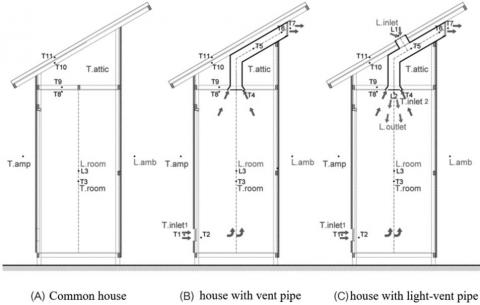Figure 3. The houses used in the experiment

Thermocouples type K (range: 0-1250 oC, accuracy ±0.5oC), heat flux sensor (Omega HFs-3, range: 1-1400 W/m2) and a pyranometer (Kipp&Zonen), Model: CMP11, range: 310-2800 µm, uncertainty<2% were connected to a data logger (Hioki: Model 8422-52, accuracy ±0.8%). The illuminance was measured by using lux meter (Testo: Model 545, accuracy ±5%). The air velocity flow was measured by using hot wire anemometer (KIMO VT-100, range: 0-50 m/s, error ±0.5%). The data interval was recorded every 30 minutes from 6:00 a.m. to 6:00 p.m.

The houses have been installed the thermocouples to measure the temperatures at the roof, attic, ceiling, room as shown in Figure 3. Further thermocouples were used to measure the inlet and outlet air temperatures, and ambient temperature. Heat flux was measured at the ceiling (room side). Air velocity was measured at the center of the pipe. The indoor illuminance and outdoor illuminance were measured on the work plane and horizontal plane, respectively.

4. Mathematical Model of the Vent Pipe

The pipe with hot air accumulating in attic area performs as air to air heat exchanger, while the heated air flows up through the pipe by buoyancy force. The house where the pipe is located has one vent on the ceiling and one vent at the overhang of the roof. The mechanism of airflow and heat transfer in the pipe can be described by mathematical models as follows:

4.1 Air flow through the pipe and heat flow rate

Illustration the average air velocity through the VP and LVP depend on the temperature difference between the inlet and outlet airflow temperature, and inlet and outlet pressure losses and wall circular friction factor.

## 4.jpg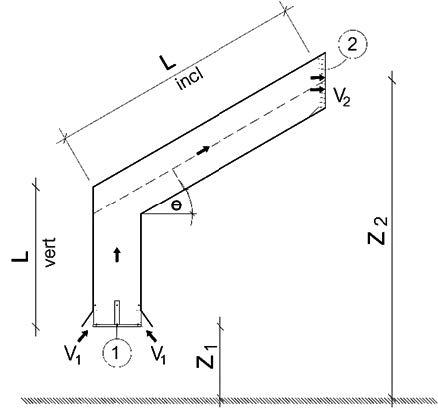Figure 4. Section the inlet and outlet air flow model

In this study, the expression for the natural flow rate by buoyancy force for study system under steady state is derived by applying Bernoulli’s equation from inlet to outlet of the VP and LVP as shown in Figure 4, The inlet and outlet air flow area is not equal the cross section area.

${{P}_{1}}+\frac{{{\rho }_{1}}v_{1}^{2}}{2}+{{\rho }_{1}}g{{Z}_{1}}={{P}_{2}}+\frac{{{\rho }_{2}}v_{2}^{2}}{2}+{{\rho }_{2}}g{{Z}_{2}}+{{\left. f\frac{L}{D}\frac{\rho {{v}^{2}}}{2} \right|}_{vert}}+{{\left. f\frac{L}{D}\frac{\rho {{v}^{2}}}{2} \right|}_{incl}}$$+{{K}_{1}}\frac{{{\rho }_{1}}v_{1}^{2}}{2}+{{K}_{30}}\frac{\rho {{v}^{2}}}{2}+{{K}_{2}}\frac{{{\rho }_{2}}v_{2}^{2}}{2} (1) \dot{m}={{\rho }_{1}}{{A}_{1}}{{v}_{1}}={{\rho }_{2}}{{A}_{2}}{{v}_{2}}=\rho {{A}_{p}}v (2) And the simplified relationship between temperature and density are given by: {{\rho }_{T}}=\rho \beta T (3) Rearrange and solve Eqns. (1), (2) and (3) obtain: \beta g(Lvert+Lincl\cdot \sin \theta )({{T}_{o}}-{{T}_{i}})=\beta {{T}_{1}}({{K}_{1}}-1)\frac{{{v}^{2}}}{2}{{\left( \frac{{{A}_{p}}}{{{A}_{1}}} \right)}^{2}}+\beta {{T}_{2}}({{K}_{2}}+1)\frac{{{v}^{2}}}{2}{{\left( \frac{{{A}_{p}}}{{{A}_{2}}} \right)}^{2}}$$+{{K}_{30}}\frac{{{v}^{2}}}{2}+\left[ {{\left. f\frac{L}{D} \right|}_{vert}}+{{\left. f\frac{L}{D} \right|}_{incl}} \right]\frac{{{v}^{2}}}{2}$    (4)

The expression of the induced air velocity is obtained by rearranging Eq. (4) yields:

${{v}^{2}}=\frac{2\beta g(Lvert+Lincl\cdot \sin \theta )({{T}_{o}}-{{T}_{i}})}{\left[ \beta {{T}_{i}}({{K}_{i}}-1){{\left( \frac{{{A}_{p}}}{{{A}_{i}}} \right)}^{2}}+\beta {{T}_{o}}({{K}_{o}}+1){{\left( \frac{{{A}_{p}}}{{{A}_{o}}} \right)}^{2}}+{{K}_{30}}+\left[ {{\left. f\frac{L}{D} \right|}_{vert}}+{{\left. f\frac{L}{D} \right|}_{incl}} \right] \right]}$     (5)

Eq. (5) gives the magnitude of the velocity induced in the pipe and together with Eq. (2), the induced mass flow rate is given by Eq. (6)

$\dot{m}={{\left( \frac{2\beta g(Lvert+Lincl\cdot \sin \theta ){{(\rho {{A}_{p}})}^{2}}({{T}_{o}}-{{T}_{i}})}{\left[ \beta {{T}_{i}}({{K}_{i}}-1){{\left( \frac{{{A}_{p}}}{{{A}_{i}}} \right)}^{2}}+\beta {{T}_{o}}({{K}_{o}}+1){{\left( \frac{{{A}_{p}}}{{{A}_{o}}} \right)}^{2}}+{{K}_{30}}+\left[ {{\left. f\frac{L}{D} \right|}_{vert}}+{{\left. f\frac{L}{D} \right|}_{incl}} \right] \right]} \right)}^{\frac{1}{2}}}$     (6)

Heat flow rate due to ventilation of air between the interior of a test house and the outside is given by Eq. (7)

${{\dot{Q}}_{vent}}=\dot{m}{{C}_{p}}({{T}_{o}}-{{T}_{i}})=\left( \rho {{C}_{p}}\frac{NV}{3600} \right)({{T}_{o}}-{{T}_{i}})$    (7)

where, f is friction coefficient (f=0.05 ), K1, K2 and K30 are inlet, outlet pressure losses and elbow pressure loss (K1=K2=3 and K30=0.2, ), Lvert is the pipe length (vertical), Lincl is pipe length (inclined), D is the diameter of the circular pipe, Ai is internal surface area of the pipe, Ko is thermal expansion coefficient, Ti is inlet air flow temperature, To is outlet air flow temperature, V is average air velocity, mo is the mass flow rate. Based on the experimental data; the inlet and outlet air flow temperature, and air velocity are used to calculate the mass flow rate in Eq. (6). These correlations will be calculated to compare the experimental data.

4.2 Heat transfer in the pipe

The problem of calculating the internal heat transfer coefficient of the pipe is reduced when the pipe surface temperature is assumed constant surface. The temperature of the air entering the pipe measured at the ceiling (Ti), the air received heat around the pipe, and the heated air flows up through the pipe by buoyancy force outgoing to the ambient as the outlet air temperature (To) as show in Figure 5. This exchange is air to air exchanger. The equations for heat exchangers applied to the pipe can be expressed as  by Eqns. (8), (9) and (10).

$\dot{m}{{C}_{p}}({{T}_{o}}-{{T}_{i}})={{h}_{i}}{{A}_{p}}\Delta {{T}_{LM}}$     (8)

${{A}_{p}}=\pi {{d}_{i}}L$    (9)

and

$\Delta {{T}_{LM}}=\frac{{{T}_{i}}-{{T}_{o}}}{\ln \left( \frac{{{T}_{s}}-{{T}_{o}}}{{{T}_{s}}-{{T}_{i}}} \right)}$     (10)

where, is the internal heat transfer coefficient, is the internal surface area of the pipe, is the log means temperature difference (LMTD) and is the pipe surface temperature. Since in this experiment LVP and VP push an accumulating heat in the attic out by transmit through the pipe surface and to the air flow.

## 5.jpg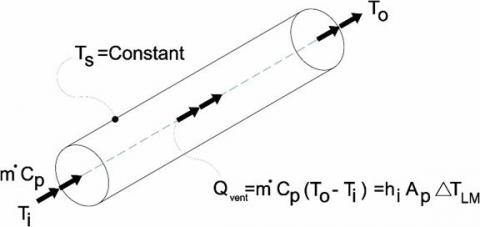Figure 5. Transmit through the pipe surface and to the air flow model

Therefore, an evaluation of internal convective heat transfer coefficient can be evaluated from energy balance. However, it can be seen that the mass flow rate (M=ρAV) changes according to the change in temperature of air that flows through LVP and VP. Finally the method is used for evaluating internal convective heat transfer coefficient to observe heat transfer ability by LVP and VP.

5. Results and Discussion

In order to evaluate the performance of the light/ vent pipe (LVP); the results are illustrated in term of temperature, ventilation, luminous flux, etc. Measurements were made of the ambient conditions such as solar radiation, ambient temperature, and wind velocity. The results are presented in two parts: the first is preliminary study between the reference house and house with vent pipe (VP), and reference house and house with light/ vent pipe (LVP). Moreover, the second is to study between a house with VP and house with LVP. During the day of testing (February 2017), there was a clear sky, the solar radiation was high, and wind velocity was very low.

5.1 Preliminary study

The preliminary study was set and carried out in order to investigate the reducing of heat accumulating in attic space using the VP and LVP compared with a reference house. The attic temperature involves to the reduction of heat transferring through the ceiling, and also induced natural air flow through the pipes. Figure 6 shows the attic temperature of the experimental houses; (a) reference house and house with VP, and (b) reference house and house with LVP.

## 6.png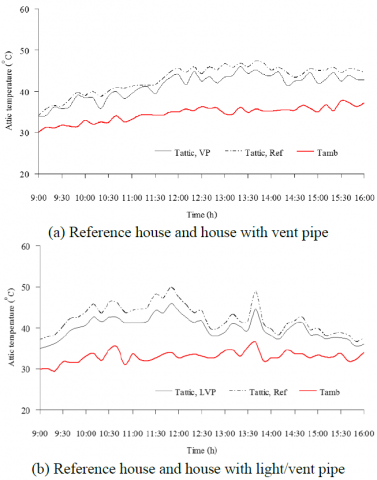Figure 6. Hourly variation of attic temperature

It had been observed that the attic temperature of VP and LVP were lower than the reference house; and also the average attic temperatures difference of (a) and (b) were calculated by measured data as about 1.61℃ and 2.51℃, respectively. According to both cases of experiments, it was found that the surfaces of VP and LVP had received heat from the attic space, while the air in the VP and LVP had been heated and driven air by buoyancy force. This behaviour is thermal exchanged air which is similar to air heat exchanger. Solar radiation from the clear sky had increased sky light transmitted through the LVP that assists producing the natural ventilation rate through the LVP, which is to be described next.

5.2 The results of VP and LVP

As previously results, in order to test the potential of VP and LVP to induce air flow through the pipes, ceiling heat gain reduction and indoor illuminance. We first show the results of measurement of the attic, inlet, and outlet air flow temperatures, and also air velocity through the pipes. Then we will illustrate the indoor illuminance through the LVP. This section is presented on a comparison between the house with VP and the house with LVP under identically ambient conditions.

5.2.1 Experiment with temperature

Experiments were conducted during daytime to record the temperatures at different positions. Three significant points were selected, which were inlet and outlet of VP and LVP, and attic of the houses. The data of these points were shown in Figures 7 and 8. The x and y axis’s of the graph depicts the hourly measured results and temperatures, respectively. Despite the similar air temperature profiles in Figure 7, it was found that the inlet air flow temperature of VP was nearly closed to the inlet air flow temperature of LVP throughout the day. There are two periods of the air flow temperature patterns: 9:00-13:00 and 13:00-15:00. The distinctive patterns of the temperatures of the inlet and outlet air flow between 13:00-15:00 in Figure 5. required a detailed examination. For more understanding, Figure 7 assists to explain the situation. Period 1: 9:00-13:00; start record at 9:00, the air in the VP and LVP received heat from the attic space and flowed through the pipes by buoyancy force, and air temperatures had increased together with the ambient temperature. It can be seen that the temperature difference between outlet and inlet air flow temperatures of LVP is higher than the VP. The reason is that LVP received a higher amount of heat, and LVP also received some sky light via a translucent flat cover on the roof. Meanwhile, Figure 7 compares the attic temperature of VP and LVP; it is apparent that the attic temperature of VP is higher than that the LVP. The highest attic temperature achieved by VP is 49℃ at 12:30, whereas 47.5℃ is achieved by LVP at the same time.

## 7.jpgFigure 7. Hourly variations of inlet and outlet air flow temperature

## 8.png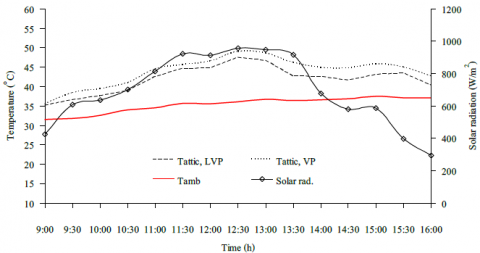Figure 8. Hourly variations of attic temperature and solar radiation

Period 2: 13:00-15:00; Figure 6 together with Figure 7 illustrate and explain the situation at the start of this period. The solar radiation had started decreasing; it is apparent that the air temperature decreased, as well as attic temperatures. Meanwhile, the inlet air flow temperature of VP and LVP decreased to nearby the ambient temperature. For this reason, the LVP can transfer more amount of heat than the VP.

5.2.2 Airflow rate of VP and LVP

The air velocity was analyzed by comparing the performance of VP and LVP in the same ambient condition. The VP and LVP are heated by heat accumulate in the attic area, and the stored heat in their fabric is utilized to induce ventilation. The pipe heated surface of both VP and LVP produces the natural ventilation that withdraws air from the room and extracts it to the ambient at the outlet area. As a consequence, outdoor air enters the room via the door opening area which makes the number of air change through the room. Applying Eqns. (5)-(7) for calculation of air velocity and volume airflow rate through the VP and LVP.

## 9.jpgFigure 9. Comparison between experimental and calculation air velocity

Figures 9 and 10 show comparisons between the measured and calculation of air velocity and volume of airflow rate through the VP and LVP in the same ambient condition. Obviously, the measured air velocity inside the LVP is much higher than that of VP. Meanwhile both of measured and calculation data show the similar trends. However, the measured data agrees reasonably well with the calculations. As expected that the volume air flow rates had fluctuated according to air velocity, then an observation shows that during 13:00-16:00 the volume air flow rate of LVP is about twice that of VP. Furthermore, the number of air change of VP and LVP were compared. Applying Eq. (7); for calculation of the number of air change by VP and LVP. It was found that the number of air change of VP and LVP were ranged 0.5-2.1 and 1-4, respectively, indicating the good performance of LVP as no moving part was used.

## 10.jpgFigure 10. Comparison between experimental and calculation volume airflow rate

5.2.3 Daylighting through the LVP

The measured illuminance for an experiment was conducted on a day in February 2017. The sky was clear whereas solar radiation was high. The amount of outdoor illuminance on a horizontal plane was about 93 kLx around noon as shown in Figure 11. The amount of daylight contribution from the ceiling of LVP on the work plane (75 cm from a floor) is ranged from 60 to 190 Lx.

## 11.jpg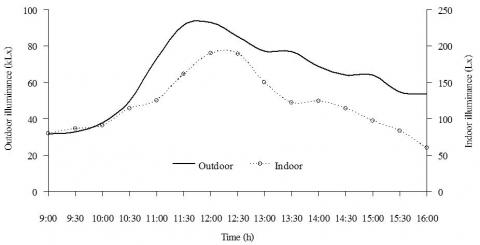Figure 11. Hourly variation of outdoor and indoor illuminance

During 9:00-12:00, the indoor illuminance increased continuously till 12:00, while from 12:00 to the evening it continuously decreased. It was apparent that the measured indoor illuminance is less than the standard indoor illuminance value (300 Lx for working plane) recommended by the Illuminance Engineering Society . However, it satisfies the recommended value for illuminance level for a place that requires about 50-150 Lx illuminance . Figure 12 shows the photographs of indoor illuminance on the maximum and minimum indoor illuminance. As in Figure 10, the minimum and maximum indoor illuminances are 190 Lx (left) and 60 Lx (right), respectively.

## 12.jpg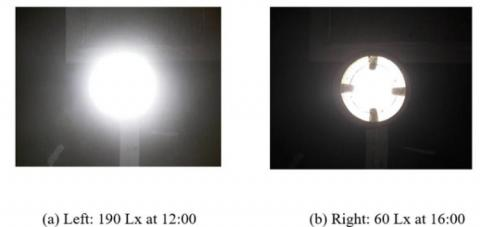Figure 12. The illuminance at the ceiling

5.2.4 Internal heat transfer in the pipe

## 13.jpg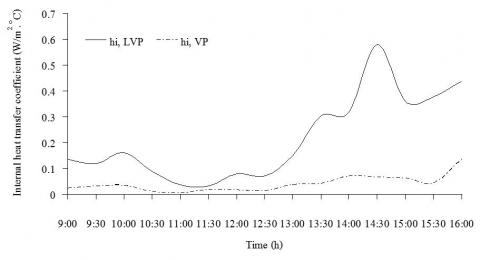Figure 13. Hourly variation of internal heat transfer coefficient

Based on the experimental data, applying the Eqns. (8) to (10) were used to calculate the internal heat transfer coefficient of LVP and VP. The internal heat transfer coefficient of LVP and VP were ranged between 0.03-0.58 W/m2-℃ and 0.005-0.1 W/m2-℃, respectively. Figure 13 together with Figure 10 assist illustrate the situation. Obviously, the internal heat transfer coefficient changes with the volume airflow rate. Apparently, the internal heat transfer coefficient of LVP is higher than the VP throughout the day, which the ranges were between 0.03-0.58 of LVP and 0.005-0.1 of VP.

5.2.5 Heat gain reduction

The heat gain has been measured at the ceiling (room side). As expected, the heat gain through the ceiling of LVP is lower than the VP because the LVP can extract heat from the attic better than the VP by natural ventilation through the pipes.

## 14.jpg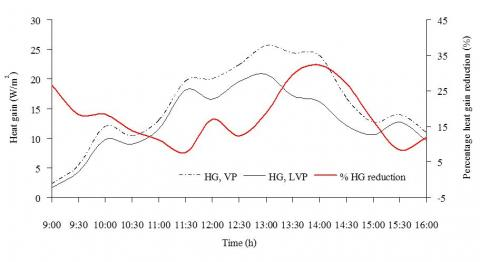Figure 14. Hourly variation of heat gain and percentage heat gain reduction

The maximum heat gain of LVP and VP are about 20.60 W/m2 and 25.60 W/m2, respectively. The maximum heat gain reduction is about 7 W/m2. The heat gain as shown in Figure 14, which can be used for calculating the percentage heat gain reduction , it is apparent that the LVP can reduce heat gain, as illustrated in term of percentage, about 8-32%.

6. Conclusions

Since LVP have a light pipe that light can pass through and make inlet air has a higher temperature than VP, the air density tends to be lower and the buoyancy force is higher than VP. Therefore, air velocity of LVP is also higher than VP which makes the convective heat transfer coefficient of LVP higher than VP. The LVP and VP had been investigated to evaluate their performances. The maximum temperature differences between the inlet and outlet temperature are 5.6oC. The highest heat gain reduction rate of LVP is about 7 W/m2 when compared to a VP. Meanwhile, the percentage of heat gain reduction which was calculated from the experimental data that shows that the reduction rate was 8 to 32%. In fact, apart from environmental and energy-saving benefits, the LVP can provide two important functions: the number of air change in the room corresponding to the induced natural ventilation rate, and sufficient day lighting without overheating. The LVP induced a higher natural ventilation rate than the VP at about 0.0002 m3/s (0.6 m3/hr). In addition, the internal heat transfer coefficient of LVP and VP were evaluated based on the experimental results, and they were varied with the volume airflow rate [26-39]. Finally, this combination concept could be applied to the other roof configurations and also modern buildings.

Acknowledgment

The authors wish to express their gratitude to Rajamangala University of Technology Rattanakosin (RMUTR) for its financial and resource support of this work Special thanks to Sunlight-Dome Co.,Ltd. for supporting the materials used in the experiment and resource support of this work and the Thailand Research Fund (contract No. RTA 5980009) and the Thailand government budget grant provided financial support for this study.

Nomenclature

A1        Inlet area of channel (m2)

A2        Outlet area of channel (m2)

Ap        Cross section area of the pipe (m2)

Cp       Specific heat at pressure constant (J/kg. °C)

F       Friction factor

G      Acceleration due gravity (m2/s)

K1       Inlet pressure loss coefficient

K2       Outlet pressure loss coefficient

K30     Elbow pressure loss coefficient

$\dot{m}$    Air mass flow rate (kg/s)

N   Number of air change (1/hr)

P1     Pressure at inlet of the pipe (Pa)

P2     Pressure at outlet of the pipe (Pa)

Q     Volume flow rate (m3/s)

v     Velocity through the cross-section area (m/s)

v1     Velocity at inlet of channel (m/s)

v2     Velocity at outlet of channel (m/s)

Greek symbols

$\theta$    Tilt angle (degree)

$\rho_{1}$    Density of air at inlet of the pipe (kg/m3)

$\rho_{2}$     Density of air at outlet of the pipe (kg/m3)

$\rho_{T}$     Density of air at any temperature (kg/m3)

$\beta$      Thermal expansion coefficient of air (1/K)

References

 Janjai, S. (2014). Solar radiation, faculty of science. Silpakorn University, p. 593.

 Khedari, J., Hirunlabh, J., Bunnag, T. (1997). Experimental study of a roof solar collector towards the natural ventilation of new houses. Energy and Buildings, 26(2): 159-164. https://doi.org/10.1016/s0378-7788(96)01030-4

 Khedari, J., Mansirisub, W., Chaima, S., Pratinthong, N., Hirunlabh, J. (2000). Field measurements of performance of roof solar collector. Energy and Buildings, 31(3): 171-178. https://doi.org/10.1016/s0378-7788(99)00003-1

 Waewsak, J., Hirunlabh, J., Khedari, J., Shin, U.C. (2003). Performance evaluation of the BSRC multi-purpose bio-climatic roof. Building and Environment, 38(11): 1297-1302. https://doi.org/10.1016/s0360-1323(03)00116-1

 Khedari, J., Ingkawanich, S., Waewsak, J., Hirunlabh, J. (2002). A PV system enhanced the performance of roof solar collector. Building and Environment, 37(12): 1317-1320. https://doi.org/10.1016/s0360-1323(01)00119-6

 Puangsombut, W., Hirunlabh, J., Khedari, J., Zeghmati, B., Win, M.M. (2007). Enhancement of natural ventilation rate and attic heat gain reduction of roof solar collector using radiant barrier. Building and Environment, 42(6): 2218-2226. https://doi.org/10.1016/j.buildenv.2005.09.028

 Khedari, J., Pongsatirat, C., Puangsombut, W., Hirunlabh, J. (2005). Experimental performance of a partially-glazed Modified Trombe Wall. International Journal of Ambient Energy, 26(1): 27-36. https://doi.org/10.1080/01430750.2005.9674968

 Ananacha, T., Puangsombut, W., Hirunlabh, J., Khedari, J. (2014). Daylighting and Thermal Performance of Thai Modern Façade Wall. Energy Procedia, 52: 271-277. https://doi.org/10.1016/j.egypro.2014.07.078

 Amornleetrakul, O., Puangsombut, W., Hirunlabh, J. (2014). Field investigation of the small house with the ventilated roof tiles. Advanced Materials Research, 931-932: 1233-1237. https://doi.org/10.4028/www.scientific.net/amr.931-932.1233

 Juengpimonyanon, K., Puangsombut, W., Ananacha, T. (2014). Field investigation on thermal performance of the tile ventilator. Applied Mechanics and Materials, 619: 73-77. https://doi.org/10.4028/www.scientific.net/amm.619.73

 Jenkins, D., Muneer, T. (2003). Modelling light-pipe performances—a natural daylighting solution. Building and Environment, 38(7): 965-972. https://doi.org/10.1016/s0360-1323(03)00061-1

 Jenkins, D., Muneer, T. (2004). Light-pipe prediction methods. Applied Energy, 79(1): 77-86. https://doi.org/10.1016/j.apenergy.2003.11.003

 Chirarattananon, S., Chedsiri, S., Renshen, L. (2000). Daylighting through light pipes in the tropics. Solar Energy, 69(4): 331-341. https://doi.org/10.1016/s0038-092x(00)00081-5

 Chirarattananon, S., Hien, V.D., Chaiwiwatworakul, P., Chirarattananon, P. (2010). Simulation of transmission of daylight through cylindrical light pipes. Sustainable Energy and Environment, 1: 97-103.

 Taengchum, T., Chirarattananon, S., Exell, R.H.B., Kubaha, K., Chaiwiwatworakul, P. (2013). A study on a ventilation stack integrated with a light pipe. Applied Thermal Engineering, 50(1): 546-554. https://doi.org/10.1016/j.applthermaleng.2012.04.045

 Yusoff, W.F.M., Salleh, E., Adam, N.M., Sapian, A.R., Yusof Sulaiman, M. (2010). Enhancement of stack ventilation in hot and humid climate using a combination of roof solar collector and vertical stack. Building and Environment, 45(10): 2296-2308. https://doi.org/10.1016/j.buildenv.2010.04.018

 Jiang, Y., Chen, Q. (2003). Buoyancy-driven single-sided natural ventilation in buildings with large openings. International Journal of Heat and Mass Transfer, 46(6): 973-988. https://doi.org/10.1016/s0017-9310(02)00373-3

 Subudhi, S., Sreenivas, K.R., Arakeri, J.H. (2013). Study of buoyant jets in natural ventilation of a model room. International Journal of Heat and Mass Transfer, 64: 91-97. https://doi.org/10.1016/j.ijheatmasstransfer.2013.04.027

 Bangalee, M.Z.I., Miau, J.J., Lin, S.Y. (2013). Computational techniques and a numerical study of a buoyancy-driven ventilation system. International Journal of Heat and Mass Transfer, 65: 572-583. https://doi.org/10.1016/j.ijheatmasstransfer.2013.06.04

 Lin, Y.J.P., Xu, Z.Y. (2013). Buoyancy-driven flows by a heat source at different levels. International Journal of Heat and Mass Transfer, 58(1-2): 312-321. https://doi.org/10.1016/j.ijheatmasstransfer.2012.11.008

 Incopera, F.P., Witt, D.D. (1981). Fundamental of Heat and Mass Transfer. Third Ed., John Wiley & Sons, United State of America.

 McQuiston, F.C., Parker, J.D., Heating, F. (1994). Ventilating and Air Conditioning Analysis and Design. Fourth Ed. John Wiley & Sons, United State of America.

 Ananacha, T., Puangsombut, W., Hirunlabh, J., Khedari, J. (2013). Field investigation of the thermal performance of a Thai modern façade Wall. International Journal of Ventilation, 12(3): 223-234. https://doi.org/10.1080/14733315.2013.11684018

 Picón-Núñez, M., Martínez-Rodríguez, G., Fuentes-Silva, A.L. (2014). Design of solar collector networks for industrial applications. Applied Thermal Engineering, 70(2): 1238-1245. https://doi.org/10.1016/j.applthermaleng.2014.05.005

 Soubdhan, T., Feuillard, T., Bade, F. (2005). Experimental evaluation of insulation material in roofing system under tropical climate. Solar Energy, 79(3): 311-320. https://doi.org/10.1016/j.solener.2004.10.009

 Prommas, R., Rattanadecho, P., Cholaseuk, D. (2010). Energy and exergy analyses in drying process of porous media using hot air. International Communications in Heat and Mass Transfer, 37(4): 372-378. https://doi.org/10.1016/j.icheatmasstransfer.2009.12.006

 Prommas, R., Keangin, P., Rattanadecho, P. (2010). Energy and exergy analyses in convective drying process of multi-layered porous packed bed. International Communications in Heat and Mass Transfer, 37(8): 1106-1114. https://doi.org/10.1016/j.icheatmasstransfer.2010.06.013

 Prommas, R., Rattanadecho, P., Jindarat, W. (2012). Energy and exergy analyses in drying process of non-hygroscopic porous packed bed using a combined multi-feed microwave-convective air and continuous belt system (CMCB). International Communications in Heat and Mass Transfer, 39(2): 242-250. https://doi.org/10.1016/j.icheatmasstransfer.2011.10.004

 Sudprasert, S., Chinsorranant, C., Rattanadecho, P. (2016). Numerical study of vertical solar chimneys with moist air in a hot and humid climate. International Journal of Heat and Mass Transfer, 102: 645-656. https://doi.org/10.1016/j.ijheatmasstransfer.2016.06.054

 Prommas, R., Phiraphat, S., Rattanadecho, P. (2019). Energy and exergy analyses of PV roof solar collector. International Journal of Heat and Technology, 37(1): 303-312. http://dx.doi.org/10.18280/ijht.370136

 Nonthiworawong, D., Rattanadecho, P., Prommas, R. (2019). Energy and exergy analysis of low-cooling in building by using light-vent pipe. Science & Technology Asia, 24(1): 41-53.

 Phongthanachote, C., Rattanadecho, P., Com-arch, C., Prommas, R. (2019). Animation and computer games design to build awareness of energy conservation. Science & Technology Asia, 24(1): 21-29.

 Naksanee, W., Prommas, R. (2018). An Experimental investigation on the efficiency of snail entry in vortex tube fed low inlet air pressure to reduce temperature of low pressure air. International Journal of Heat and Technology 36(4): 1223-1232. http://dx.doi.org/10.18280/ijht.360409

 Rattanapunt, P., Com-arch, C., Sungsoontorn, S., Prommas, R. (2018). A study of characteristics of palm oil biomass by using torrefaction process. Science & Technology Asia, 23(4): 23-31.

 Sriphan, U., Kerdchang, P., Prommas, R., Bunnang, T. (2018). Coefficient of performance of battery running and charging by magnet generator Bedini. Journal of Electrochemical Energy Conversion and Storage, 15(4): 041002. https://doi.org/10.1115/1.4039504

 Phiraphat, S., Prommas, R., Puangsombut, W. (2017). Experimental study of natural convection in PV roof solar collector. International Communications in Heat and Mass Transfer, 89: 31-38. https://doi.org/10.1016/j.icheatmasstransfer.2017.09.022

 Prommas, R., Rungsakthaweekul, T. (2014). Effect of microwave curing conditions on high strength concrete properties. Energy Procedia, 56: 26-34. https://doi.org/10.1016/j.egypro.2014.07.128

 Prommas, R., Runngsakthaweekul, T., Minabhun, N. (2018). Recycled of asphalt road using microwave heating. Thaksin University Journal, 21(2): 69-77.

 Prommas, R., Runngsakthaweekul, T., Kesawadkorn, P. (2017). Mechanical properties of porous concrete with effective micro-organisms (EM) mixed. Thaksin University Journal, 20(2): 74-83.